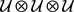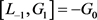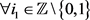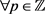﻿ 一类超Heisenberg-Virasoro代数的超双代数结构

# 一类超Heisenberg-Virasoro代数的超双代数结构Lie Super-Bialgebra Structures on a Super Heisenberg-Virasoro Algebra

Abstract: In this paper we investigate Lie super-bialgebra structures on a super Heisenberg-Virasoro algebra. We obtain sufficient and necessary conditions for this type Lie super-bialgebra structures to be triangular coboundary.

1. 引言

$\begin{array}{l}\left[{L}_{m},{L}_{n}\right]=\left(m-n\right){L}_{m+n},\text{\hspace{0.17em}}\text{\hspace{0.17em}}\left[{L}_{m},{I}_{n}\right]=-n{I}_{m+n},\text{\hspace{0.17em}}\text{\hspace{0.17em}}\left[{L}_{m},{G}_{n}\right]=-n{G}_{m+n},\\ \left[{I}_{n},{G}_{n}\right]=\left[{I}_{m},{I}_{n}\right]=0,\text{\hspace{0.17em}}\text{\hspace{0.17em}}\left[{G}_{m},{G}_{n}\right]={I}_{m+n}\end{array}$ (1.1)

2. 预备知识

$\phi \left({L}^{i},{L}^{j}\right)\subset {L}^{i+j}$$\text{Ker}\left(1-\tau \right)\subset \text{Ker}\phi$$\phi \cdot \left(1\otimes \phi \right)\cdot \left(1+\xi +{\xi }^{2}\right)=0$

$\Delta \left({L}^{i}\right)\subset \underset{j+k=i}{\sum }{L}^{j}\otimes {L}^{k}$$\mathrm{Im}\Delta \subset \mathrm{Im}\left(1-\tau \right)$$\left(1+\xi +{\xi }^{2}\right)\cdot \left(1\otimes \Delta \right)\cdot \Delta =0$

$x\cdot \left(\underset{i}{\sum }{a}_{i}\otimes {b}_{i}\right)=\underset{i}{\sum }\left(\left[x,{a}_{i}\right]\otimes {b}_{i}+{\left(-1\right)}^{|x||{a}_{i}|}{a}_{i}\otimes \left[x,{b}_{i}\right]\right)$ (2.1)

$\mathcal{U}$ 表示L的泛包络代数，记 $r=\underset{i}{\sum }{a}_{i}\otimes {b}_{i}\in L\otimes L$，引入的元素

${r}^{13}=\underset{i}{\sum }{a}_{i}\otimes 1\otimes {b}_{i}=\left(\tau \otimes 1\right)\left(1\otimes r\right)=\left(1\otimes \tau \right)\left(r\otimes 1\right)$

${r}^{12}=\underset{i}{\sum }{a}_{i}\otimes {b}_{i}\otimes 1=r\otimes 1$${r}^{23}=\underset{i}{\sum }1\otimes {a}_{i}\otimes {b}_{i}=1\otimes r$ . (2.2)

$c\left(r\right)=\left[{r}^{12},{r}^{13}\right]+\left[{r}^{12},{r}^{23}\right]+\left[{r}^{13},{r}^{23}\right]$$\forall r\in L\otimes L$ .

2) 我们称 $\left(L,\phi ,\Delta ,r\right)$ 为三角的，若满足经典的Yang-Baxter方程 (CYBE)

$c\left(r\right)=0$ (2.3)

3) 元素 $r\in \text{Im}\left(1-\tau \right)\subset L\otimes L$ 称为满足修正的Yang-Baxter方程，如果

$x\cdot c\left(r\right)=0$$\forall x\in L$ (2.4)

$r\in \text{Im}\left(1-\tau \right)\subset L\otimes L$，则有 $\left(1+\xi +{\xi }^{2}\right)\cdot \left(1\otimes {\Delta }_{r}\right)\cdot {\Delta }_{r}\left(x\right)=x\cdot c\left(r\right)$，三元组 $\left(L,\left[\cdot ,\cdot \right],{\Delta }_{r}\right)$ 是李双代数当且仅当r满足(2.3)。

$D\left(\left[x,y\right]\right)={\left(-1\right)}^{|D||x|}x\cdot D\left(y\right)-{\left(-1\right)}^{|y|\left(|D|+|x|\right)}y\cdot D\left(x\right)$$\forall x,y\in \mathcal{L}$ (2.5)

$\mathcal{D}\left({L}_{0}\right)\equiv 0\equiv \mathcal{D}\left({I}_{\text{0}}\right)$$\mathcal{D}\left({I}_{n}\right)=2\nu \left({I}_{0}\otimes {I}_{n}-{I}_{n}\otimes {I}_{0}\right)$$\forall n\in {ℤ}^{*}$$m\in ℤ$ ,

$\mathcal{D}\left({G}_{m}\right)=\nu \left({I}_{0}\otimes {G}_{m}-{G}_{m}\otimes {I}_{0}\right)+{\nu }^{\prime }\left({I}_{m}\otimes {G}_{0}-{G}_{0}\otimes {I}_{m}\right)+\omega \left({I}_{0}\otimes {I}_{m}-{I}_{m}\otimes {I}_{0}\right)$ ,

$\mathcal{D}\left({L}_{n}\right)=\left(\left(2-n\right)\lambda +\left(n-1\right)\eta \right){I}_{n}\otimes {I}_{0}+\left(\left(n-2\right)\lambda +\frac{2-n}{2}\eta +\frac{n}{2}\rho \right){I}_{0}\otimes {I}_{n}$ . (2.6)

$\mathcal{D}\left({L}_{0}\right)\equiv 0$ 表示 $\mathcal{D}\left({L}_{0}\right)\equiv 0\left(\mathrm{mod}ℂ\left({I}_{0}\otimes {I}_{0}\right)\right)$，即 $\mathcal{D}\left({L}_{0}\right)\in ℂ\left({I}_{0}\otimes {I}_{0}\right)$

3. 主要结果及证明过程

${D}_{0}\left({L}_{n}\right)=\underset{i\in ℤ}{\sum }\left({a}_{n,i}{L}_{i}\otimes {L}_{n-i}+{b}_{n,i}{L}_{i}\otimes {I}_{n-i}+{b}_{n,i}^{†}{I}_{i}\otimes {L}_{n-i}+{c}_{n,i}{I}_{i}\otimes {I}_{n-i}+{e}_{n,i}{G}_{i}\otimes {G}_{n-i}\right)$

${D}_{0}\left({I}_{n}\right)=\underset{i\in ℤ}{\sum }\left({\alpha }_{n,i}{L}_{i}\otimes {L}_{n-i}+{\beta }_{n,i}{L}_{i}\otimes {I}_{n-i}+{\beta }_{n,i}^{†}{I}_{i}\otimes {L}_{n-i}+{\gamma }_{n,i}{I}_{i}\otimes {I}_{n-i}+{f}_{n,i}{G}_{i}\otimes {G}_{n-i}\right)$

${D}_{0}\left({G}_{n}\right)=\underset{i\in ℤ}{\sum }\left({\mu }_{n,i}{L}_{i}\otimes {G}_{n-i}+{\mu }_{n,i}^{†}{G}_{i}\otimes {L}_{n-i}+{\nu }_{n,i}{I}_{i}\otimes {G}_{n-i}+{\nu }_{n,i}^{†}{G}_{i}\otimes {I}_{n-i}\right)$

${L}_{1}\cdot \left({L}_{n}\otimes {L}_{-n}\right)=\left(1-n\right){L}_{n+1}\otimes {L}_{-n}+\left(1+n\right){L}_{n}\otimes {L}_{1-n}$

${L}_{1}\cdot \left({I}_{n}\otimes {I}_{-n}\right)=-n{I}_{n+1}\otimes {I}_{-n}+n{I}_{n}\otimes {I}_{1-n}$${L}_{1}\cdot \left({G}_{n}\otimes {G}_{-n}\right)=-n{G}_{n+1}\otimes {G}_{-n}+n{G}_{n}\otimes {G}_{1-n}$

${L}_{1}\cdot \left({L}_{n}\otimes {I}_{-n}\right)=\left(1-n\right){L}_{n+1}\otimes {I}_{-n}+n{L}_{n}\otimes {I}_{1-n}$${L}_{1}\cdot \left({I}_{n}\otimes {L}_{-n}\right)=-n{I}_{n+1}\otimes {L}_{-n}+\left(1+n\right){I}_{n}\otimes {L}_{1-n}$

${D}_{0}-{u}_{\text{inn}}$ 代替 ${D}_{0}$，其中u是 ${L}_{p}\otimes {L}_{-p}$${L}_{p}\otimes {I}_{-p}$${I}_{p}\otimes {L}_{-p}$${I}_{p}\otimes {I}_{-p}$${G}_{p}\otimes {G}_{-p}$ ( $p\in ℤ$ )的适当的线性组合，假设对任意 $i\in ℤ\\left\{-1,2\right\}$$j\in ℤ\\left\{0,2\right\}$$k\in ℤ\\left\{-1,1\right\}$$m\in ℤ\\left\{0,1\right\}$，有 ${a}_{1,i}={b}_{1,j}={b}_{1,k}^{†}={c}_{1,m}={e}_{1,m}=0$。则 ${D}_{0}\left({L}_{1}\right)$ 化简为

$\begin{array}{c}{D}_{0}\left({L}_{1}\right)={a}_{1,-1}{L}_{-1}\otimes {L}_{2}+{a}_{1,2}{L}_{2}\otimes {L}_{-1}+{b}_{1,0}{L}_{0}\otimes {I}_{1}+{b}_{1,2}{L}_{2}\otimes {I}_{-1}+{b}_{1,-1}^{†}{I}_{-1}\otimes {L}_{\text{\hspace{0.17em}}2}\\ \text{\hspace{0.17em}}\text{\hspace{0.17em}}+{b}_{1,1}^{†}{I}_{1}\otimes {L}_{0}+{c}_{1,0}{I}_{0}\otimes {I}_{1}+{c}_{1,1}{I}_{1}\otimes {I}_{0}+{e}_{1,0}{G}_{0}\otimes {G}_{1}+{e}_{1,1}{G}_{1}\otimes {G}_{0}\end{array}$ .

${D}_{0}$ 作用在 $\left[{L}_{-1},{L}_{1}\right]=-2{L}_{0}$ 上，有

${a}_{-1,i}=0$$\forall \text{ }i\in ℤ\\left\{-2,±1,0\right\}$${a}_{-1,-2}=-{a}_{1,-1}+\frac{1}{3}{a}_{-1,0}$${a}_{-1,1}=-{a}_{1,2}-\frac{1}{3}{a}_{-1,0}$(3.1)

${b}_{-1,{i}_{1}}={b}_{-1,{i}_{\text{2}}}^{†}={c}_{-1,{i}_{\text{3}}}={e}_{-1,{i}_{\text{3}}}=0$$\forall {i}_{\text{1}}\in ℤ\\left\{±1,0\right\}$${i}_{\text{2}}\in ℤ\\left\{-2,-1,0\right\}$$\text{ }{i}_{\text{3}}\in ℤ\\left\{-1,0\right\}$

${b}_{1,0}={b}_{1,2}={b}_{1,-1}^{†}={b}_{1,1}^{†}=\text{0}$${b}_{-1,0}=-2{b}_{-1,-1}=-2{b}_{-1,1}$${b}_{-1,-1}^{†}=-2{b}_{-1,-2}^{†}=-2{b}_{-1,0}^{†}$ .

$\begin{array}{c}{D}_{0}\left({L}_{-1}\right)={a}_{-1,-2}{L}_{-2}\otimes {L}_{1}+{a}_{-1,0}\left(-{L}_{-1}\otimes {L}_{0}+{L}_{0}\otimes {L}_{-1}\right)+{a}_{-1,1}{L}_{1}\otimes {L}_{-2}\\ \text{\hspace{0.17em}}\text{\hspace{0.17em}}+{b}_{-1,-1}\left({L}_{-1}\otimes {I}_{0}-2{L}_{0}\otimes {I}_{-1}+{L}_{1}\otimes {I}_{-2}\right)\\ \text{\hspace{0.17em}}\text{\hspace{0.17em}}+{b}_{-1,0}^{†}\left({I}_{-2}\otimes {L}_{1}-2{I}_{-1}\otimes {L}_{0}+{I}_{0}\otimes {L}_{-1}\right)\\ \text{\hspace{0.17em}}\text{\hspace{0.17em}}+{c}_{-1,-1}{I}_{-1}\otimes {I}_{0}+{c}_{-1,0}{I}_{0}\otimes {I}_{-1}+{e}_{-1,-1}{G}_{-1}\otimes {G}_{0}+{e}_{-1,0}{G}_{0}\otimes {G}_{-1}\end{array}$

${D}_{0}\left({L}_{1}\right)={a}_{1,-1}{L}_{-1}\otimes {L}_{2}+{a}_{1,2}{L}_{2}\otimes {L}_{-1}+{c}_{1,0}{I}_{0}\otimes {I}_{1}+{c}_{1,1}{I}_{1}\otimes {I}_{0}+{e}_{1,0}{G}_{0}\otimes {G}_{1}+{e}_{1,1}{G}_{1}\otimes {G}_{0}$ .

${D}_{0}$ 作用在 $\left[{L}_{-2},{L}_{1}\right]=-3{L}_{-1}$ 上，结合(3.1)得

${a}_{-1,1}=-\frac{1}{3}{a}_{-1,0}$$2{a}_{-2,-1}+3{a}_{-2,0}-3{a}_{-1,0}=0$${a}_{-2,0}+4{a}_{-2,1}-3{a}_{-1,1}=0$(3.2)

${a}_{-1,-2}=\frac{1}{3}{a}_{-1,0}$$4{a}_{-2,-3}+{a}_{-2,-2}-3{a}_{-1,-2}=0$$3{a}_{-2,-2}+2{a}_{-2,-1}+3{a}_{-1,0}=0$ . (3.3)

${D}_{0}$ 作用在 $\left[{L}_{-1},{L}_{2}\right]=-3{L}_{1}$ 上，有

${a}_{-1,0}={a}_{2,-1}-{a}_{2,3}={a}_{2,0}+4{a}_{2,3}={a}_{2,1}-6{a}_{2,3}={a}_{2,2}+4{a}_{2,3}={a}_{2,i}={b}_{2,{i}_{1}}={b}_{2,{i}_{2}}^{†}={c}_{2,{i}_{3}}={e}_{2,{i}_{3}}=0$

$\forall i\in ℤ\\left\{±1,0,2,3\right\}$${i}_{1}\in ℤ\\left\{±1,0,2,3\right\}$${i}_{2}\in ℤ\\left\{±1,0,2,3\right\}$${i}_{3}\in ℤ\\left\{0,1,2\right\}$

${b}_{2,0}+\text{3}{b}_{2,-1}={b}_{2,3}-{b}_{-1,-1}={b}_{2,1}-3{b}_{2,-1}-{b}_{-1,-1}=3{b}_{2,2}+{b}_{2,1}+5{b}_{-1,-1}=0$(3.4)

$3{b}_{2,3}^{†}+{b}_{2,2}^{†}={b}_{2,-1}^{†}-{b}_{-1,0}^{†}={b}_{2,1}^{†}+3{b}_{2,0}^{†}+5{b}_{-1,0}^{†}=-3{b}_{2,3}^{†}+{b}_{2,1}^{†}-{b}_{-1,0}^{†}=0$ . (3.5)

${D}_{0}$ 作用在 $\left[{L}_{-2},{L}_{2}\right]=-4{L}_{0}$ 上，结合(3.4)和(3.5)得，则有

${a}_{2,3}+{a}_{-2,1}={c}_{2,1}+{c}_{-2,-1}={e}_{2,1}+{e}_{-2,-1}=0$

${b}_{2,-1}={b}_{-2,0}=0$${b}_{-2,-1}=-3{b}_{2,1}=-3{b}_{-1,-1}$${b}_{-2,-2}=-{b}_{2,2}=2{b}_{-1,-1}$${b}_{-2,1}={b}_{-1,-1}$

${b}_{-2,-2}^{†}={b}_{2,3}^{†}=0$${b}_{-2,-1}^{†}=-3{b}_{2,1}^{†}=-3{b}_{-1,0}^{†}$${b}_{-2,0}^{†}=-{b}_{2,0}^{†}=2{b}_{-1,0}^{†}$${b}_{-2,-3}^{†}={b}_{-1,0}^{†}$ .

${u}_{1}={L}_{1}\otimes {L}_{-1}-2{L}_{0}\otimes {L}_{0}+{L}_{-1}\otimes {L}_{1}$${u}_{2}={L}_{1}\otimes {I}_{-1}-{L}_{0}\otimes {I}_{0}$${u}_{3}={I}_{-1}\otimes {L}_{1}-{I}_{0}\otimes {L}_{0}$，用 ${D}_{0}-{a}_{2,3}{\left({u}_{1}\right)}_{\text{inn}}-{b}_{-1,-1}{\left({u}_{2}\right)}_{\text{inn}}-{b}_{-1,0}^{†}{\left({u}_{3}\right)}_{\text{inn}}$ 代替D，有 ${a}_{2,3}={b}_{-1,-1}={b}_{-1,0}^{†}=0$。则有

${D}_{0}\left({L}_{±1}\right)={c}_{±1,0}{I}_{0}\otimes {I}_{±1}+{c}_{±1,±1}{I}_{±1}\otimes {I}_{0}+{e}_{±1,0}{G}_{0}\otimes {G}_{±1}+{e}_{±1,±1}{G}_{±1}\otimes {G}_{0}$

${D}_{0}\left({L}_{±2}\right)={c}_{±2,0}{I}_{0}\otimes {I}_{±2}±{c}_{2,1}{I}_{±1}\otimes {I}_{±1}+{c}_{±2,±2}{I}_{±2}\otimes {I}_{0}+{e}_{±2,0}{G}_{0}\otimes {G}_{±2}±{e}_{2,1}{G}_{±1}\otimes {G}_{±1}+{e}_{±2,±2}{G}_{±2}\otimes {G}_{0}$ .

${c}_{-1,-1}+{c}_{-1,0}+{c}_{1,0}+{c}_{1,1}={c}_{-2,-2}+{c}_{-2,0}+{c}_{2,0}+{c}_{2,2}=2{c}_{2,0}+{c}_{2,1}+{c}_{-1,0}-3{c}_{1,0}=0$

$2{c}_{2,2}+{c}_{2,1}+{c}_{-1,-1}-3{c}_{1,1}=2{c}_{-2,0}-{c}_{2,1}+{c}_{1,0}-3{c}_{-1,0}=2{c}_{-2,-2}-{c}_{2,1}+{c}_{1,1}-3{c}_{-1,-1}=0$ .

${D}_{0}$ 作用在 $\left[{L}_{-1},{I}_{1}\right]=-{I}_{0}$ 上，有

${\alpha }_{1,i}={\beta }_{1,{i}_{1}}={\beta }_{1,{i}_{2}}^{†}={\gamma }_{1,{i}_{3}}={f}_{1,{i}_{3}}={\alpha }_{1,1}+3{\alpha }_{1,2}={\alpha }_{1,0}-3{\alpha }_{1,2}={\alpha }_{1,-1}+{\alpha }_{1,2}=0$

$\forall i\in ℤ\\left\{±1,0,2\right\}$${i}_{\text{1}}\in ℤ\\left\{±1,0\right\}$${i}_{\text{2}}\in ℤ\\left\{0,1,2\right\}$${i}_{\text{3}}\in ℤ\\left\{0,1\right\}$

${\beta }_{1,0}=-2{\beta }_{1,-1}=-2{\beta }_{1,1}$${\beta }_{1,1}^{†}=-2{\beta }_{1,0}^{†}=-2{\beta }_{1,2}^{†}$${\gamma }_{1,0}+{\gamma }_{1,1}={f}_{1,0}+{f}_{1,1}=0$ .

${D}_{0}$ 作用在 $\left[{L}_{1},{I}_{-1}\right]={I}_{0}$ 上，同理有

$\begin{array}{c}{D}_{0}\left({I}_{-1}\right)={\alpha }_{-1,1}\left(-{L}_{-2}\otimes {L}_{1}+3{L}_{-1}\otimes {L}_{0}-3{L}_{0}\otimes {L}_{-1}+{L}_{1}\otimes {L}_{-2}\right)\\ \text{\hspace{0.17em}}\text{\hspace{0.17em}}+{\beta }_{-1,1}\left({L}_{-1}\otimes {I}_{0}-2{L}_{0}\otimes {I}_{-1}+{L}_{1}\otimes {I}_{-2}\right)\\ \text{\hspace{0.17em}}\text{\hspace{0.17em}}+{\beta }_{-1,0}^{†}\left({I}_{-2}\otimes {L}_{1}-2{I}_{-1}\otimes {L}_{0}+{I}_{0}\otimes {L}_{-1}\right)\\ \text{\hspace{0.17em}}\text{\hspace{0.17em}}+{\gamma }_{-1,-1}\left({I}_{-1}\otimes {I}_{0}-{I}_{0}\otimes {I}_{-1}\right)+{f}_{-1,-1}\left({G}_{-1}\otimes {G}_{0}-{G}_{0}\otimes {G}_{-1}\right)\end{array}$ .

${D}_{0}$ 作用在 $\left[{L}_{2},{I}_{-1}\right]={I}_{1}$ 上，有

${\alpha }_{-1,1}={\alpha }_{1,2}={\beta }_{-1,1}={\beta }_{1,1}={\beta }_{-1,0}^{†}={\beta }_{1,2}^{†}=0$${\gamma }_{1,0}+{\gamma }_{-1,-1}={f}_{1,0}+{f}_{-1,-1}=0$ .

${D}_{0}$ 作用在 $\left[{L}_{1},{G}_{\text{0}}\right]=0$$\left[{L}_{-1},{G}_{0}\right]=0$ 上，可得

${D}_{0}\left({G}_{0}\right)={\nu }_{0,0}{I}_{0}\otimes {G}_{0}+{\nu }_{0,0}^{†}{G}_{0}\otimes {I}_{0}$

${D}_{0}\left({L}_{±1}\right)={c}_{±1,0}{I}_{0}\otimes {I}_{±1}+{c}_{±1,±1}{I}_{±1}\otimes {I}_{0}$ .

${D}_{0}$ 作用在 $\left[{I}_{-1},{G}_{\text{1}}\right]=0$$\left[{I}_{1},{G}_{-\text{1}}\right]=0$ 上，分别有以下系数关系式

${\mu }_{1，1}={\mu }_{1,-1}^{†}=-{\mu }_{1,2}=-{\mu }_{1,0}^{†}={f}_{1,0}$${\mu }_{\text{1},{i}_{\text{1}}}={\mu }_{\text{1},{i}_{\text{2}}}^{†}=0$$\forall {i}_{\text{1}}\in ℤ\\left\{1,2\right\}$${i}_{\text{2}}\in ℤ\\left\{-1,0\right\}$

${\mu }_{-1,-2}={\mu }_{-1,0}^{†}=-{\mu }_{-1,-1}=-{\mu }_{-1,1}^{†}={f}_{1,0}$${\mu }_{-1,{i}_{\text{3}}}={\mu }_{-1,{i}_{\text{4}}}^{†}=0$$\forall {i}_{\text{3}}\in ℤ\\left\{-2,-1\right\}$${i}_{\text{4}}\in ℤ\\left\{0,\text{1}\right\}$ .

${D}_{0}$ 作用在 $\left[{L}_{1},{G}_{-1}\right]={G}_{0}$上，我们可以推导出

${f}_{1,0}={\nu }_{1,{i}_{\text{1}}}={\nu }_{1,{i}_{\text{1}}}^{†}={\nu }_{1,1}+{\nu }_{1,0}-{\nu }_{0,0}={\nu }_{1,1}^{†}+{\nu }_{1,0}^{†}-{\nu }_{0,0}^{†}=0$${\nu }_{-1,{i}_{2}}={\nu }_{-1,{i}_{2}}^{†}={\nu }_{-1,-1}+{\nu }_{-1,0}-{\nu }_{0,0}={\nu }_{-1,-1}^{†}+{\nu }_{-1,0}^{†}-{\nu }_{0,0}^{†}=0$$\forall {i}_{\text{2}}\in ℤ\\left\{-1,0\right\}$ .

${D}_{0}$ 作用在 $\left[{L}_{\text{2}},{G}_{-1}\right]={G}_{\text{1}}$$\left[{L}_{-\text{2}},{G}_{\text{1}}\right]=-{G}_{-1}$ 上，可得 ${e}_{2,0}={e}_{2,1}={e}_{2,2}=0$

${D}_{0}$ 作用在 $\left[{G}_{-\text{1}},{G}_{\text{1}}\right]={I}_{0}$ 上，有

${D}_{0}\left({G}_{0}\right)={\nu }_{0,0}\left({I}_{0}\otimes {G}_{0}-{G}_{0}\otimes {I}_{0}\right)$

${D}_{0}\left({G}_{±1}\right)={\nu }_{1,0}\left({I}_{0}\otimes {G}_{±1}-{G}_{±1}\otimes {I}_{0}\right)+{\nu }_{1,1}\left({I}_{±1}\otimes {G}_{0}-{G}_{0}\otimes {I}_{±1}\right)$

${D}_{0}\left({L}_{0}\right)\equiv 0\equiv {D}_{0}\left({I}_{\text{0}}\right)$${D}_{0}\left({I}_{n}\right)=2\nu \left({I}_{0}\otimes {I}_{n}-{I}_{n}\otimes {I}_{0}\right)$

${D}_{0}\left({L}_{n}\right)=\left(\left(2-n\right)\lambda +\left(n-1\right)\eta \right){I}_{n}\otimes {I}_{0}+\left(\left(n-2\right)\lambda +\frac{2-n}{2}\eta +\frac{n}{2}\rho \right){I}_{0}\otimes {I}_{n}$

${D}_{0}\left({G}_{m}\right)=\nu \left({I}_{0}\otimes {G}_{m}-{G}_{m}\otimes {I}_{0}\right)+{\nu }^{\prime }\left({I}_{m}\otimes {G}_{0}-{G}_{0}\otimes {I}_{m}\right)$$\forall n\in {ℤ}^{*}$$\text{ }m\in ℤ$ .

${D}_{0}\left({L}_{n}\right)=\underset{i\in ℤ}{\sum }\left({a}_{n,i}{L}_{i}\otimes {G}_{n-i}+{a}_{n,i}^{†}{G}_{i}\otimes {L}_{n-i}+{b}_{n,i}{I}_{i}\otimes {G}_{n-i}+{b}_{n,i}^{†}{G}_{i}\otimes {I}_{n-i}\right)$

${D}_{0}\left({I}_{n}\right)=\underset{i\in ℤ}{\sum }\left({\alpha }_{n,i}{L}_{i}\otimes {G}_{n-i}+{\alpha }_{n,i}^{†}{G}_{i}\otimes {L}_{n-i}+{\beta }_{n,i}{I}_{i}\otimes {G}_{n-i}+{\beta }_{n,i}^{†}{G}_{i}\otimes {I}_{n-i}\right)$

${D}_{0}\left({G}_{n}\right)=\underset{i\in ℤ}{\sum }\left({\mu }_{n,i}{L}_{i}\otimes {L}_{n-i}+{\nu }_{n,i}{L}_{i}\otimes {I}_{n-i}+{\nu }_{n,i}^{†}{I}_{i}\otimes {L}_{n-i}+{\omega }_{n,i}{I}_{i}\otimes {I}_{n-i}+{\lambda }_{n,i}{G}_{i}\otimes {G}_{n-i}\right)$

${L}_{1}\cdot \left({L}_{n}\otimes {G}_{-n}\right)=\left(1-n\right){L}_{n+1}\otimes {G}_{-n}+n{L}_{n}\otimes {G}_{1-n}$${L}_{1}\cdot \left({I}_{n}\otimes {G}_{-n}\right)=-n{I}_{n+1}\otimes {G}_{-n}+n{I}_{n}\otimes {G}_{1-n}$

${L}_{1}\cdot \left({G}_{n}\otimes {L}_{-n}\right)=-n{G}_{n+1}\otimes {L}_{-n}+\left(n+1\right){G}_{n}\otimes {L}_{1-n}$${L}_{1}\cdot \left({G}_{n}\otimes {I}_{-n}\right)=-n{G}_{n+1}\otimes {I}_{-n}+n{G}_{n}\otimes {I}_{1-n}$ .

${D}_{0}-{u}_{\text{inn}}$ 代替 ${D}_{0}$，其中u是 ${L}_{p}\otimes {G}_{-p}$${G}_{p}\otimes {L}_{-p}$${I}_{p}\otimes {G}_{-p}$${G}_{p}\otimes {I}_{-p}$ 的适当的线性组合，，假设对于任意 $i\in ℤ\\left\{0,2\right\}$$j\in ℤ\\left\{-1,1\right\}$$k\in ℤ\\left\{0,1\right\}$，有 ${a}_{1,i}={a}_{1,i}^{†}={b}_{1,k}={b}_{1,k}^{†}=0$。则 ${D}_{0}\left({L}_{1}\right)$ 可写成

$\begin{array}{c}{D}_{0}\left({L}_{1}\right)={a}_{1,\text{0}}{L}_{\text{0}}\otimes {G}_{1}+{a}_{1,2}{L}_{2}\otimes {G}_{-1}+{a}_{1,-1}^{†}{G}_{-1}\otimes {L}_{2}+{a}_{1,1}^{†}{G}_{1}\otimes {L}_{0}\\ \text{\hspace{0.17em}}\text{\hspace{0.17em}}+{b}_{1,0}{I}_{0}\otimes {G}_{1}+{b}_{1,1}{I}_{1}\otimes {G}_{0}+{b}_{1,0}^{†}{G}_{0}\otimes {I}_{1}+{b}_{1,1}^{†}{G}_{1}\otimes {I}_{0}\end{array}$ .

${D}_{0}$ 作用在 $\left[{L}_{-1},{L}_{1}\right]=-2{L}_{0}$ 上，记 ${u}_{\text{1}}=-{L}_{0}\otimes {G}_{0}+{L}_{1}\otimes {G}_{-1}$${u}_{\text{2}}={G}_{-1}\otimes {L}_{1}-{L}_{\text{0}}\otimes {G}_{\text{0}}$，用 ${D}_{0}-{a}_{-1,-1}{\left({u}_{1}\right)}_{\text{inn}}-{a}_{-1,0}^{†}{\left({u}_{2}\right)}_{\text{inn}}$ 代替D，可得

${D}_{0}\left({L}_{±\text{1}}\right)={b}_{±1,0}{I}_{0}\otimes {G}_{±1}+{b}_{±1,±1}{I}_{±1}\otimes {G}_{0}+{b}_{±1,0}^{†}{G}_{0}\otimes {I}_{±1}+{b}_{±1,±1}^{†}{G}_{±1}\otimes {I}_{0}$ .

${D}_{0}$ 依次作用在 $\left[{L}_{-2},{L}_{1}\right]=-3{L}_{-1}$$\left[{L}_{-1},{L}_{2}\right]=-3{L}_{1}$$\left[{L}_{-2},{L}_{2}\right]=-4{L}_{0}$ 上，

$\begin{array}{c}{D}_{0}\left({L}_{±2}\right)={b}_{±2,\text{0}}{I}_{0}\otimes {G}_{±2}±{b}_{2,1}{I}_{±1}\otimes {G}_{±1}+{b}_{±2,±2}{I}_{±2}\otimes {G}_{0}\\ \text{\hspace{0.17em}}\text{\hspace{0.17em}}+{b}_{±2,\text{0}}^{†}{G}_{\text{0}}\otimes {I}_{±\text{2}}±{b}_{2,1}^{†}{G}_{±1}\otimes {I}_{±1}+{b}_{±2,±\text{2}}^{†}{G}_{±\text{2}}\otimes {I}_{\text{0}}\end{array}$ .

$2{b}_{2,0}^{†}+{b}_{2,1}^{†}+{b}_{-1,0}^{†}-3{b}_{1,0}^{†}=2{b}_{2,2}^{†}+{b}_{2,1}^{†}+{b}_{-1,-1}^{†}-3{b}_{1,1}^{†}=0$(3.6)

$2{b}_{2,0}+{b}_{2,1}+{b}_{-1,1}-3{b}_{1,0}=\text{2}{b}_{2,2}+{b}_{2,\text{1}}+{b}_{-1,-1}-3{b}_{1,1}=2{b}_{-2,0}^{†}-{b}_{2,1}^{†}+{b}_{1,0}^{†}-3{b}_{-1,0}^{†}=0$ (3.7)

$2{b}_{-2,-2}-{b}_{2,1}+{b}_{\text{1},1}-3{b}_{-1,-1}=\text{2}{b}_{-2,\text{0}}-{b}_{2,\text{1}}+{b}_{1,0}-3{b}_{-1,0}=2{b}_{-2,-2}^{†}-{b}_{2,1}^{†}+{b}_{1,1}^{†}-3{b}_{-1,-1}^{†}=0$ .(3.8)

${D}_{0}$ 依次作用在 $\left[{L}_{-1},{I}_{1}\right]=-{I}_{0}$$\left[{L}_{\text{1}},{I}_{-1}\right]={I}_{0}$$\left[{L}_{2},{I}_{-1}\right]={I}_{1}$ 上，我们可将 ${D}_{0}\left({I}_{±1}\right)$ 化简为

${D}_{0}\left({I}_{±1}\right)={\beta }_{1,0}\left({I}_{0}\otimes {G}_{±1}-{I}_{±1}\otimes {G}_{0}\right)+{\beta }_{1,0}^{†}\left({G}_{0}\otimes {I}_{±1}-{G}_{±1}\otimes {I}_{0}\right)$ .

${D}_{0}$ 作用在 $\left[{L}_{±1},{G}_{0}\right]=0$$\left[{I}_{-1},{G}_{0}\right]=0$ 上，可得

${D}_{0}\left({G}_{0}\right)={\omega }_{0,0}{I}_{0}\otimes {I}_{0}+{\lambda }_{0,0}{G}_{0}\otimes {G}_{0}$ .

${D}_{0}$ 作用在 $\left[{L}_{±1},{G}_{\mp 1}\right]=±{G}_{0}$$\left[{L}_{2},{G}_{-1}\right]={G}_{1}$ 上，再结合(3.6)~(3.8)，可以推导出

${D}_{0}\left({L}_{±1}\right)={D}_{0}\left({L}_{±2}\right)={D}_{0}\left({G}_{0}\right)=0$

${D}_{\text{0}}\left({G}_{±1}\right)={\omega }_{1,0}\left({I}_{0}\otimes {I}_{±1}-{I}_{±1}\otimes {I}_{0}\right)+{\lambda }_{1,0}\left({G}_{0}\otimes {G}_{±1}-{G}_{±1}\otimes {G}_{0}\right)$ .

${D}_{0}$ 作用在 $\left[{I}_{-\text{1}},{G}_{1}\right]=\text{0}$ 上，有 ${D}_{0}\left({I}_{±1}\right)=0$

${D}_{0}$ 作用在 $\left[{G}_{-\text{1}},{G}_{1}\right]={I}_{0}$ 上，有 ${D}_{0}\left({G}_{±1}\right)={\omega }_{1,0}\left({I}_{0}\otimes {I}_{±1}-{I}_{±1}\otimes {I}_{0}\right)$

${D}_{0}\left({G}_{0}\right)={D}_{0}\left({L}_{m}\right)={D}_{0}\left({I}_{m}\right)=0$${D}_{0}\left({G}_{n}\right)=\omega \left({I}_{0}\otimes {I}_{n}-{I}_{n}\otimes {I}_{0}\right)$$\forall m\in ℤ$$n\in {ℤ}^{*}$ .

$\left(\mathcal{L},\left[\cdot ,\cdot \right],\Delta \right)$$\mathcal{L}$ 上的超李双代数结构。由定理3.1得，在(2.6)中提到对某个 $r\in \mathcal{L}\otimes \mathcal{L}$，有 $\Delta ={\Delta }_{r}$ 当且仅当 $\lambda =\eta =\rho =\omega =\nu ={\nu }^{\prime }=0$。结合 $\mathrm{Im}\Delta \subset \mathrm{Im}\left(1-\tau \right)$ 和引理3.2，可以推导出对某个 $c\in ℂ$，有 $r-c{I}_{0}\otimes {I}_{0}\in \text{Im}\left(1-\tau \right)$，则引理3.1得出 $c\left(r\right)\in ℂ{I}_{0}\otimes {I}_{0}$。因此， $\left(\mathcal{L},\left[\cdot ,\cdot \right],\Delta \right)$ 是一个三角余边缘的超李双代数当且仅当 $\lambda =\eta =\rho =\omega =\nu ={\nu }^{\prime }=0$。故定理3.2得证。

 Drinfeld, V.G. (1983) Constant Quasiclassical Solutions of the Yang-Baxter Quantum Equation. Soviet Mathematics Doklady, 28, 667-671.

 Belavin, A.A. and Drinfeld, V.G. (1982) Solutions of the Classical Yang-Baxter Equation for Simple Lie Algebras. Functional Analysis and Its Applications, 16, 159-180.
https://doi.org/10.1007/BF01081585

 Michaelis, W. (1994) A Class of Infinite-Dimensional Lie Bialgebras Containing the Virasoro Algebras. Advances in Mathematics, 107, 365-392.
https://doi.org/10.1006/aima.1994.1062

 Taft, E.J. (1993) Witt and Virasoro Algebras as Lie Bialgebras. Journal of Pure and Applied Algebra, 87, 301-312.
https://doi.org/10.1016/0022-4049(93)90116-B

 Ng, S.H. and Taft, E.J. (2000) Classification of the Lie Bialgebra Structures on the Witt and Virasoro Algebras. Journal of Pure and Applied Algebra, 151, 67-88.
https://doi.org/10.1016/S0022-4049(99)00045-6

 Han, J.Z., Li, J.B. and Su, Y.C. (2009) Lie Bialgebra Structures on the Schrödinger-Virasoro Lie Algebra. Journal of Mathematical Physics, 50, Article ID: 083504.
https://doi.org/10.1063/1.3187784

 Song, G.A. and Su, Y.C. (2006) Lie Bialgebras of Generalized Witt Type. Science in China: Series A, 49, 533-544.
https://doi.org/10.1007/s11425-006-0533-7

 Wu, Y.Z., Song, G.A. and Su, Y.C. (2006) Lie Bialgebras of Generalized Virasoro-Like Type. Acta Mathematica Sinica, English Series, 22, 1915-1922.
https://doi.org/10.1007/s10114-005-0742-y

Top